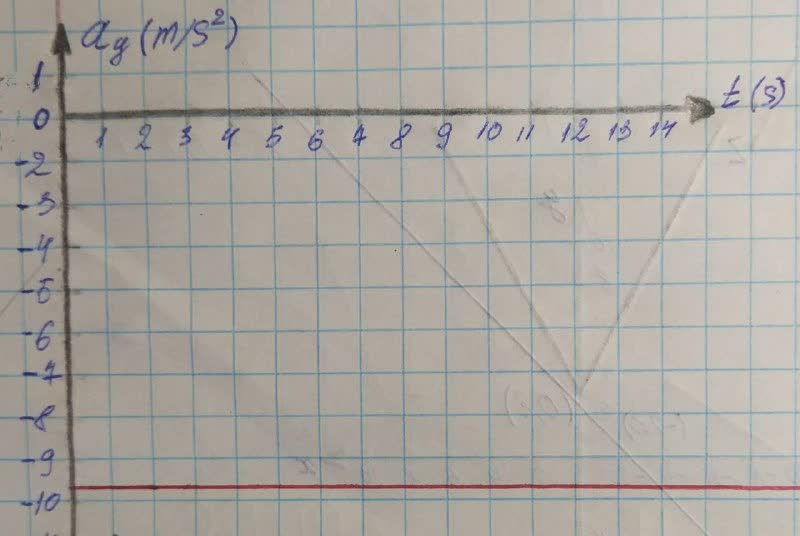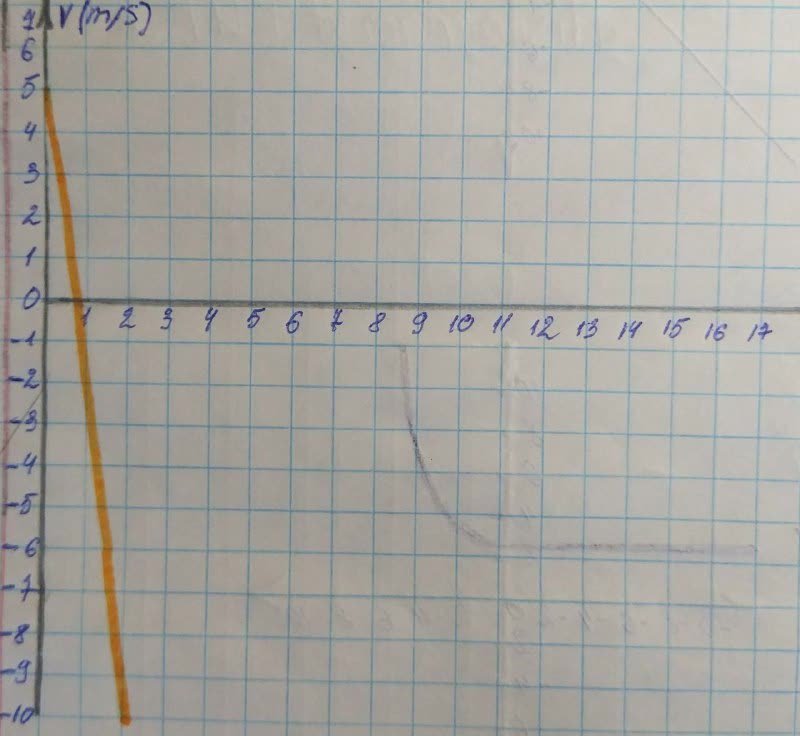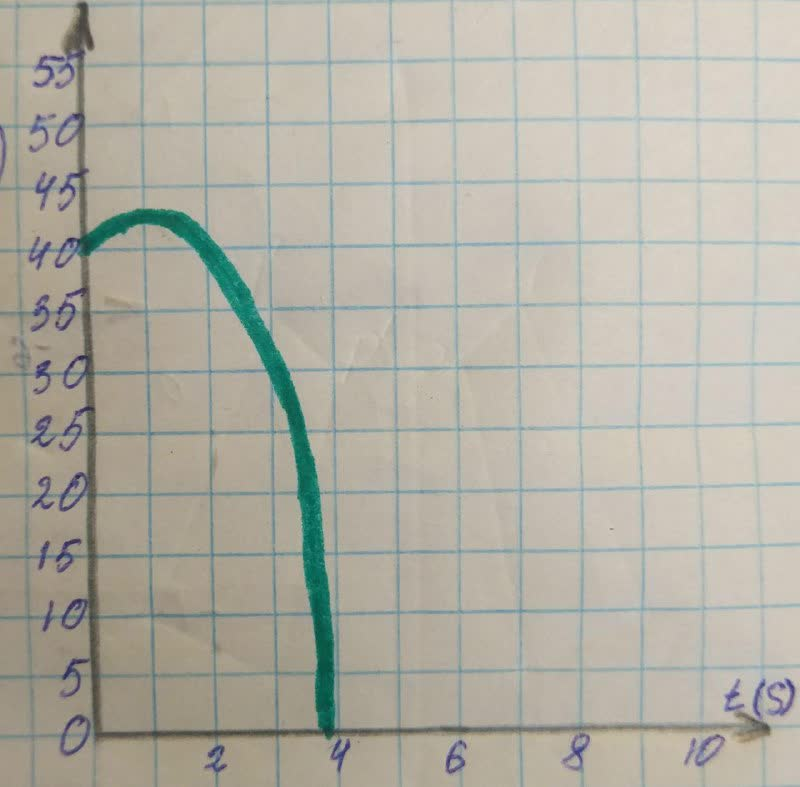# A hot-air balloonist, rising vertically with a constant velocity of magnitude 5.Bobbie Comstock 2021-12-17 Answered

A hot-air balloonist, rising vertically with a constant velocity of magnitude 5.00 m/s, releases a sandbag at an instant when the balloon is 40.0 m above the ground. After the sandbag is released, it is in free fall. (a) Compute the position and velocity of the sandbag at 0.250 s and 1.00 s after its release. (b) How many seconds after its release does the bag strike the ground? (c) With what magnitude of velocity does it strike the ground? (d) What is the greatest height above the ground that the sandbag reaches? (e) Sketch ${a}_{y}-t,{v}_{y}t$, and y-t graphs for the motion.

You can still ask an expert for help

• Live experts 24/7
• Questions are typically answered in as fast as 30 minutes
• Personalized clear answers

Solve your problem for the price of one coffee

• Math expert for every subject
• Pay only if we can solve itFasaniu

Step 1
1Concepts and Principles
1- Particle under constant acceleration: If a particle moves in a straight line with a constant acceleration ${a}_{x}$, its motion is described by kinematics equations, from which we will use the following equation:
(1)${v}_{xf}={v}_{xi}+{a}_{y}t$
(2)${x}_{f}={x}_{i}+{v}_{\xi }t+\frac{1}{2}{a}_{x}{t}^{2}$
2- The sulution to a quadration in the from: $a{x}^{2}+bx+x=0$ is given by the quadrat formula:
$x=\frac{-b±\sqrt{{b}^{2}-4ac}}{2a}$
Step 2
2 Given Data
Let the +y-direction be upwards and the initial position of the sand bag be at ${y}_{i}=0$.
${v}_{yi}\text{(initial velocity of the bag)}=5\frac{m}{s}$
${y}_{f}\text{(position of the bag when it reaches the ground)}=-40m$
${a}_{y}\text{(acceleration due to gravity)}=-9.8\frac{m}{{s}^{2}}$
Step 3
3 Required Data
In part (a), we are asked to find the position and the velocity of the sandbag at t=0.25 s and t=1 s.
In part(b), we are asked to find the time taken by the bag to reach the ground.
In part(c), we are asked to find the speed with which the bag strikes the ground.
In part(d), we are asked to find the maximum height the bag reaches above the ground.
In part(e), we are asked to sketch task graphs for the motion of the sand bag.
Step 4
4 Solution
(a)
Model the sand bag as a particle under constant acceleration and apply Equations (1) and (2) to find the final velocity and final position of the sand bag as a function of time:
${v}_{yf}={v}_{yi}+{a}_{y}t$
${y}_{f}={y}_{i}+{v}_{yi}t+\frac{1}{2}{a}_{y}{t}^{2}$
For t=0.25 s:
${v}_{yf}=5\frac{m}{s}+\left(-9.8\frac{m}{{s}^{2}}\right)\left(0.25s\right)=2.55\frac{m}{s}$
${y}_{f}=0+\left(5\frac{m}{s}\right)\left(0.25s\right)+\frac{1}{2}\left(-9.8\frac{m}{{s}^{2}}\right){\left(0.25s\right)}^{2}=0.944m$
Therefore, at t=0.25 s, the sand bag is moving with an upward velocity of magnitude 2.55 m/s and is 40 m+0.944 m=40.9 m above the ground.
For t=0.1 s:
${v}_{yf}=5\frac{m}{s}+\left(-9.8\frac{m}{{s}^{2}}\right)\left(1s\right)=-4.8\frac{m}{s}$
${y}_{f}=0+\left(5\frac{m}{s}\right)\left(1s\right)+\frac{1}{2}\left(-9.8\frac{m}{{s}^{2}}\right){\left(1s\right)}^{2}=0.1m$
Therefore, at t=1 s, the sand bag is moving with an downward velocity of magnitude 4.8 m/s and is 40 m+0.1 m=40.1 m above the ground.

We have step-by-step solutions for your answer!Rita Miller

Step 5
(b)
The sand bag strikes the ground when its final position is ${y}_{f}=-40m$. From Equation (2):
${y}_{f}={y}_{i}+{v}_{yit}+\frac{1}{2}{a}_{y}{t}^{2}$
$-40m=0+\left(5\frac{m}{s}\right)t+\frac{1}{2}\left(-9.8\frac{m}{{s}^{2}}\right){t}^{2}$
$-40m=\left(5\frac{m}{s}\right)t-\left(4.9\frac{m}{{s}^{2}}\right){t}^{2}$
$\left(4.9m{s}^{2}\right){t}^{2}-\left(5\frac{m}{s}\right)t-40m=0$
Solve this equation for t using the quadratic formula from Equation (3), for :
$t=\frac{-b±\sqrt{{b}^{2}-4ac}}{2a}$
$t=\frac{-\left(-5\frac{m}{s}\right)±\sqrt{{\left(-5\frac{m}{s}\right)}^{2}-4\left(4.9\frac{m}{{s}^{2}}\right)\left(-40m\right)}}{2\left(4.9\frac{m}{{s}^{2}}\right)}$
=3.41 s or -2.39 s
A negative value for time is phisically unacceptable. Therefore, the time take for the sand bag to reach the ground is t=3.41 s.
Step 6
(c)
The velocity of the bag before it atrikes the ground is fround from Equation (l):
${v}_{yf}={v}_{yi}+{a}_{y}t$
Where t is the time taken for the sand bag to reach the ground which we found in part (b). Subsitute numerical values:
${v}_{yf}=5\frac{m}{s}+\left(-9.8\frac{m}{{s}^{2}}\right)\left(3.41s\right)=-28.4\frac{m}{s}$
The negative values indicates that the direction of the velocity ia downwads.
Step 7
(d)
The bag reaches maximum heightwhen its final velosity ${v}_{yf}=0$. From Equation (2), we find the time taken by the bag to reach this maximum height:
${v}_{yf}={v}_{yi}+{a}_{y}t$

$t=\frac{5\frac{m}{s}}{9.8\frac{m}{{s}^{2}}}=0.51s$
They by using Equation (2), we can find the maximum height:
$y={y}_{0}+{v}_{yi}t+\frac{1}{2}{a}_{y}{t}^{2}$
$y={0}_{5\frac{m}{s}}\left(0.51s\right)+\frac{1}{2}\left(-9.8\frac{m}{{s}^{2}}\right){\left(0.51s\right)}^{2}$
y=1.28 m
Therefore, the maximum height of the sand bag is 40 m+1.28 m=41.3 m.

We have step-by-step solutions for your answer!Don Sumner

Step 8
(e)
The ${a}_{y}-t$ graph is a horithontal line since the acceleration is constant at ${a}_{y}=-9.8m/{s}^{2}$Step 9
The velosity oa the sand bag is a function of time from Eguation (l) as follows:
${v}_{y}={v}_{yi}+{a}_{y}t=5m/s-\left(9.8m/{s}^{2}\right)t$
Therefore, the ${v}_{y}-t$ graphs looks like:Step 10
The y - t graph look like (let y=0 at the ground level):Result
(a)At t=0.25 s, the sand bag is moving with an upward velocity of magnitude 2.55 m/s and is 40.9 m above the ground. At t=1 s, the sand bag is moving with an downwoard velosity of magnitude 4.8 m/s and is 40.1 m above the ground.
(b)t=3.41 s
(c)${v}_{yt}=-28.4$ m/s
(d)The maximum height of the sand is 41.3 m.
(e)Click for graphs

We have step-by-step solutions for your answer!